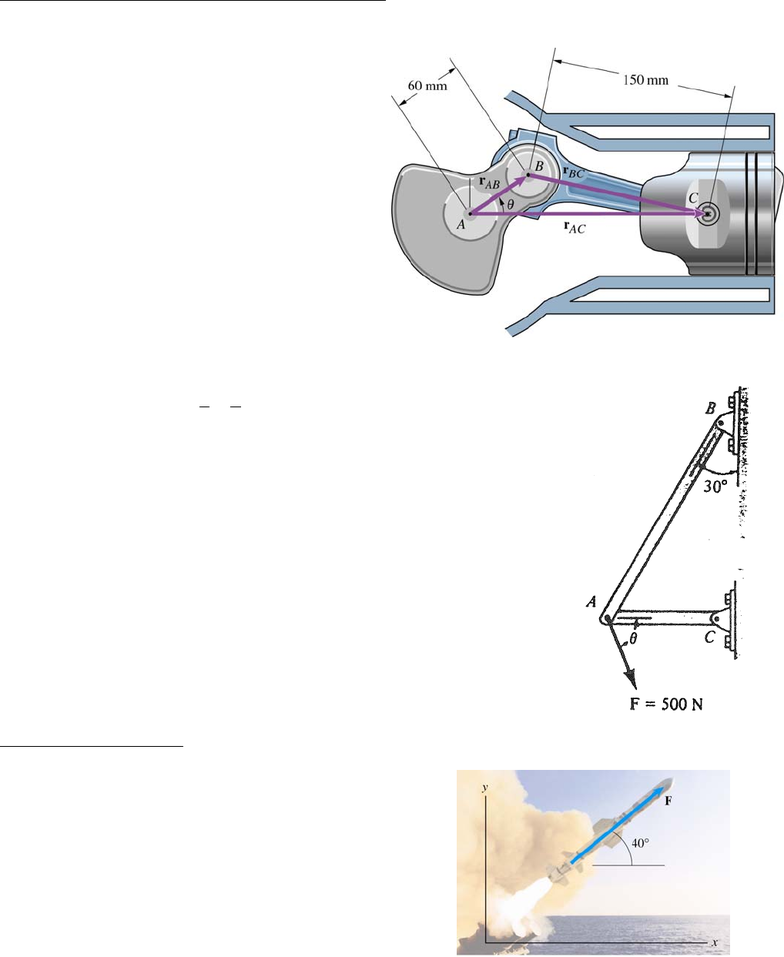# ENGG 202 Lecture Notes - Lecture 1: Resultant Force, Toyota Electronic Modulated Suspension

89 views3 pagesENGG 202 Tutorial 2 January 15/16, 2013
1) The angle θ = 30o. What is the magnitude of the vector rAC? ANS: rAC = 199 mm
Solution Strategy: vector triangle is drawn for
you, use trig (cosine law) to solve for the unknown
magnitude
2) The force F acting on the frame shown has a magnitude of 500 N and is
to be resolved into two components acting along struts AB and AC.
Determine the angle θ (0o < θ < 90o) so that the component FAC is directed
from A towards C and has a magnitude of 400 N. ANS: 76.1o
Solution Strategies: Directions of the components along AB and AC are
known. Draw a force triangle adding vectors tip to tail with the 500 N force
being the resultant. One component is the 400 N force (tail of 400 N
connects to tail of resultant). Other component is added to 400 N vector
and is parallel to line AB. Use trig to solve for the unknown forces/angles.
Rectangular components:
3) The missile’s engine exerts a 260 kN force F. Express F in terms of components using the
coordinate system shown. ANS: 199i + 167j (kN)
Solution Strategy: use trig to find the x and y components
of the force
Unlock document

This preview shows page 1 of the document.
Unlock all 3 pages and 3 million more documents.